# Resistance Measurement

Here is a problem that was recently sent to me: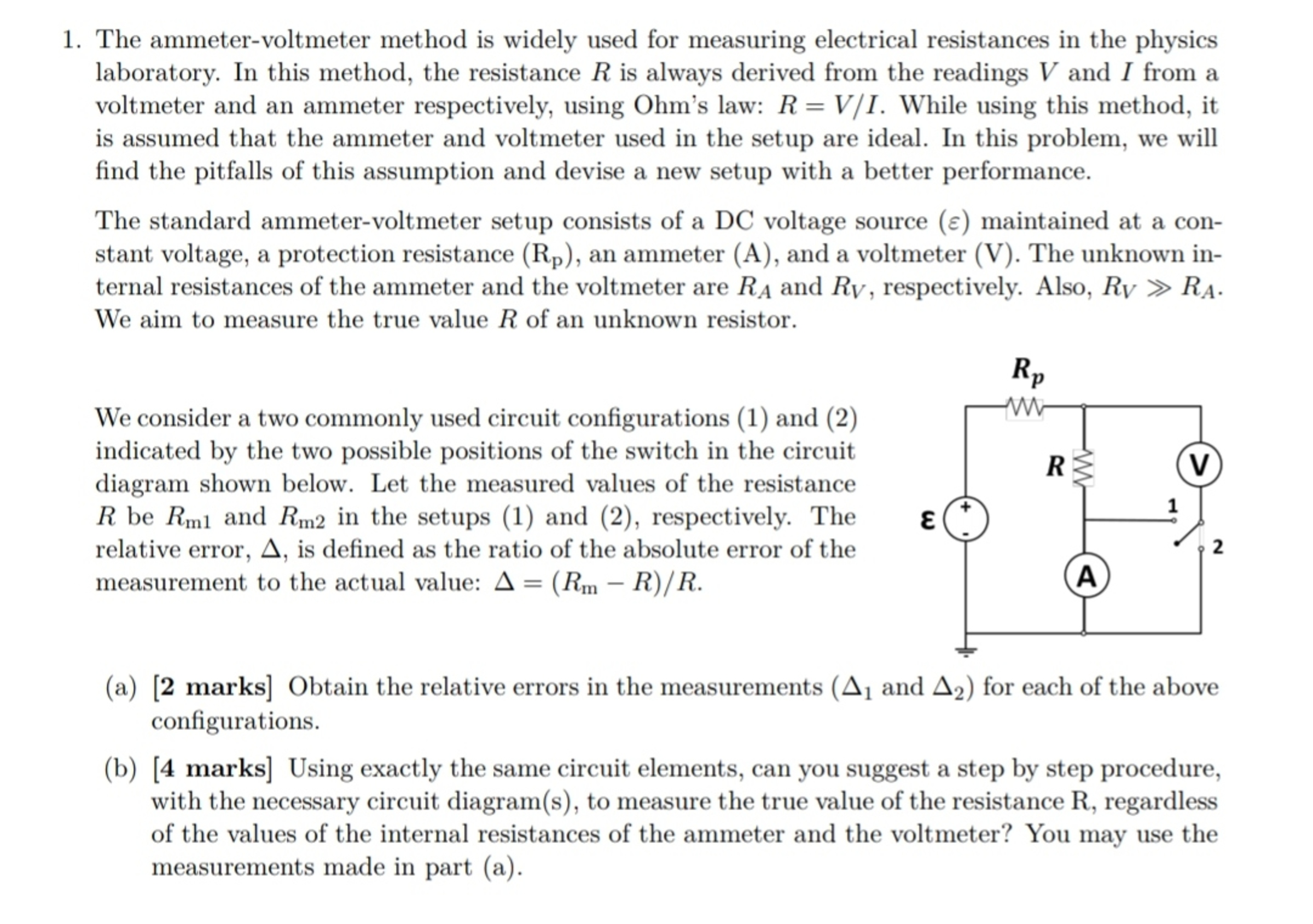To solve, I will refer to the following four configurations. The first two are the ones presented in the problem (with the switches in different state), and the third and fourth are more useful configurations.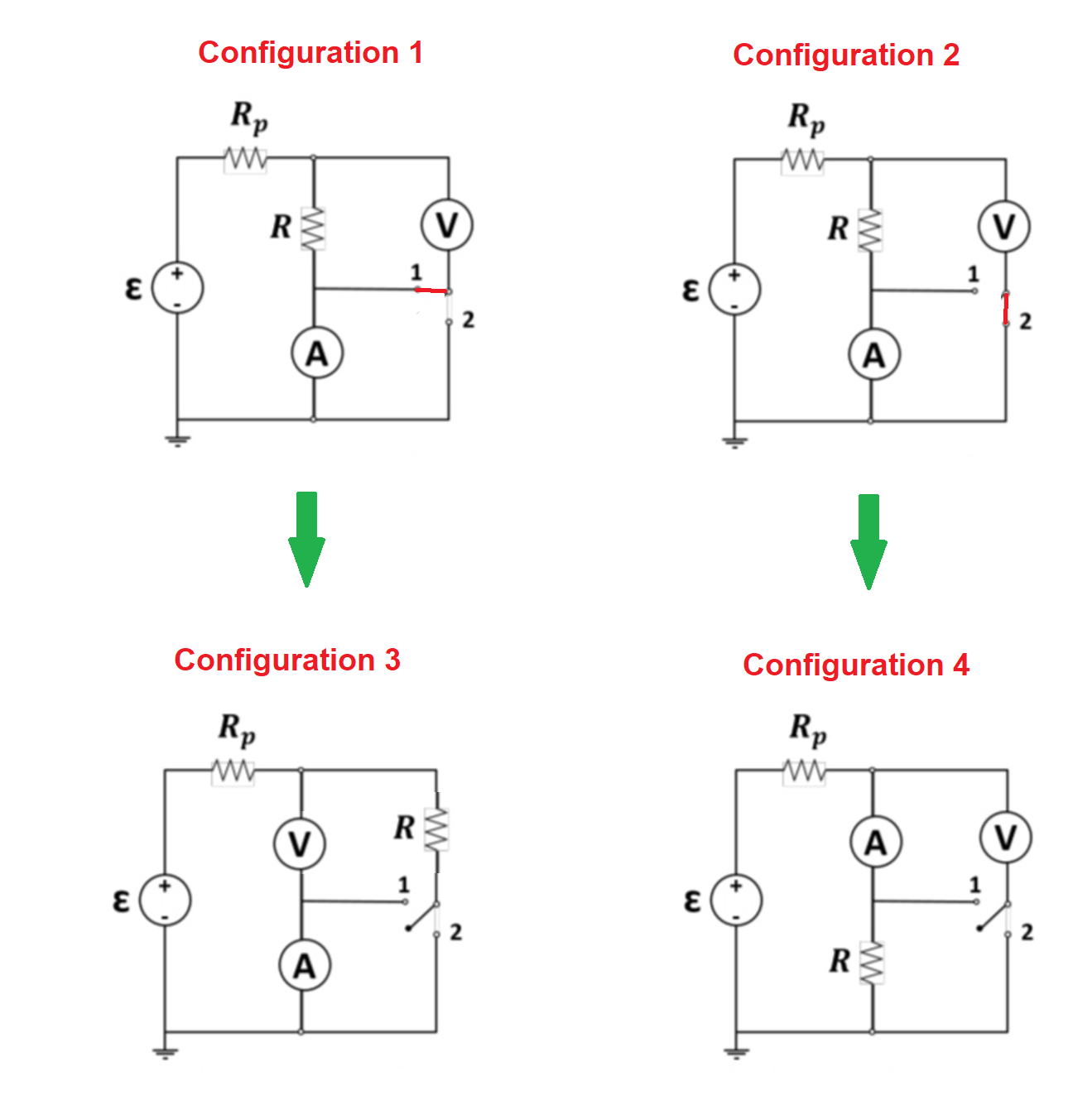Configuration 1:

The voltage across the voltmeter can be expressed in terms of the current through the ammeter:

$V = I \frac{R R_v}{R + R_v}$

The measured resistance in configuration 1 is therefore:

$R_{m1} =\frac{V}{I} = \frac{R R_v }{R + R_v} \\ \Delta_1 = \frac{R_v }{R + R_v} - 1 = -\frac{R}{R + R_v}$

Configuration 2:

The current through the ammeter can be expressed in terms of the voltage across the voltmeter:

$I = \frac{V}{R + R_A}$

The measured resistance in configuration 2 is therefore:

$R_{m2} = \frac{V}{I} = R + R_A \\ \Delta_2 = 1 + \frac{R_A}{R} - 1 = \frac{R_A}{R}$

There are two ways to answer part (b) of the question: using either configuration 3 or configuration 4.

Configuration 3:

Configuration 3 is a more useful form of configuration 1. Starting with the switch in position 2, we know the current through $R_v$ as well as the voltage across it, which gives us $R_v$. Then with the switch in position 1, we get $R_m = \frac{R R_v }{R + R_v}$ (which is the same result from configuration 1). And since the first measurement gave us $R_v$, we can solve for $R$.

Configuration 4:

Configuration 4 is a more useful form of configuration 2. Starting with the switch in position 1, we know the current through $R_A$ as well as the voltage across it, which gives us $R_A$. Then with the switch in position 2, we get $R_m = R + R_A$ (which is the same result from configuration 2). And since the first measurement gave us $R_A$, we can solve for $R$.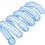Note by Steven Chase
6 months, 1 week ago

This discussion board is a place to discuss our Daily Challenges and the math and science related to those challenges. Explanations are more than just a solution — they should explain the steps and thinking strategies that you used to obtain the solution. Comments should further the discussion of math and science.

When posting on Brilliant:

• Use the emojis to react to an explanation, whether you're congratulating a job well done , or just really confused .
• Ask specific questions about the challenge or the steps in somebody's explanation. Well-posed questions can add a lot to the discussion, but posting "I don't understand!" doesn't help anyone.
• Try to contribute something new to the discussion, whether it is an extension, generalization or other idea related to the challenge.

MarkdownAppears as
*italics* or _italics_ italics
**bold** or __bold__ bold
- bulleted- list
• bulleted
• list
1. numbered2. list
1. numbered
2. list
Note: you must add a full line of space before and after lists for them to show up correctly
paragraph 1paragraph 2

paragraph 1

paragraph 2

[example link](https://brilliant.org)example link
> This is a quote
This is a quote
    # I indented these lines
# 4 spaces, and now they show
# up as a code block.

print "hello world"
# I indented these lines
# 4 spaces, and now they show
# up as a code block.

print "hello world"
MathAppears as
Remember to wrap math in $$ ... $$ or $ ... $ to ensure proper formatting.
2 \times 3 $2 \times 3$
2^{34} $2^{34}$
a_{i-1} $a_{i-1}$
\frac{2}{3} $\frac{2}{3}$
\sqrt{2} $\sqrt{2}$
\sum_{i=1}^3 $\sum_{i=1}^3$
\sin \theta $\sin \theta$
\boxed{123} $\boxed{123}$

Sort by:

@Steven Chase i have posted a new discussion on mechanis. Please solve that problem.

- 3 months, 1 week ago

- 3 months, 1 week ago

At last did you get any quadratic equation for $R$ which you have not written in your solution ?

- 6 months, 1 week ago

This is the first problem of InPho!

- 6 months, 1 week ago

Did I get it right?

- 6 months, 1 week ago

I think you did!, I had no clue about how to do the second part, and spent a lot of time getting an accurate error for the first part (some current goes into the voltmeter, that thought messed me up, I ended up obtaining a huge expression for error even after using binomial approximations multiple times)

- 6 months, 1 week ago

The problem doesn't do a great job explaining what assumptions you are allowed to make. That annoyed me a bit

- 6 months, 1 week ago

I assume minimal assumptions was best, also could I ask you the solution for another problem of the same paper

- 6 months, 1 week ago

I can take a look, but I can't promise that I'll be able to solve it

- 6 months, 1 week ago

Thank you for trying, even if you get it or not (I hope you do)

- 6 months, 1 week ago

OK, it's just about bed time on my side of the planet, so I'll try tomorrow.

- 6 months, 1 week ago

It’s fine even if you give the answer a week later

- 6 months, 1 week ago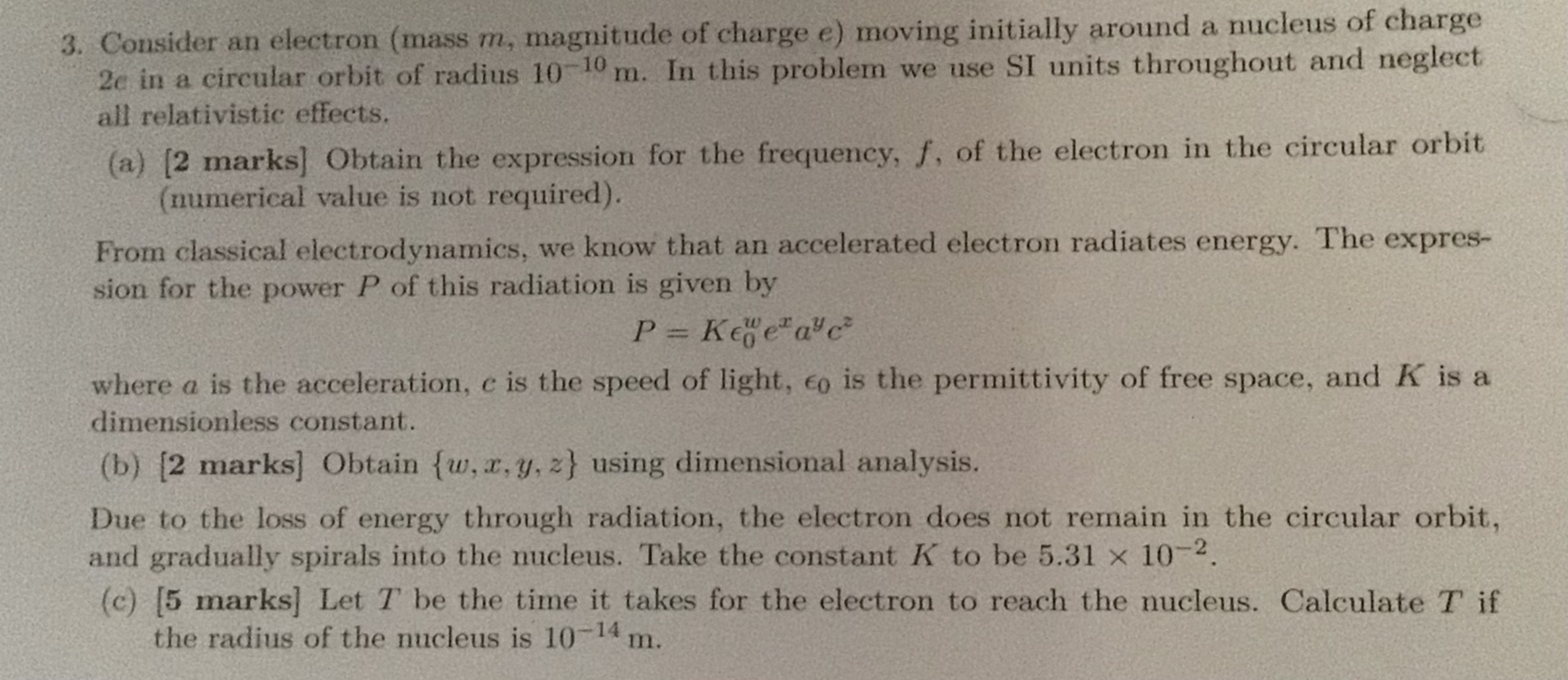I assumed this would be easy, but got stuck in middle when I had to perform an integration between time and distance, I am now unclear of how to approach this now

- 6 months, 1 week ago

@Jason Gomez I think you have probably stucked in part (c).
Here is the solution: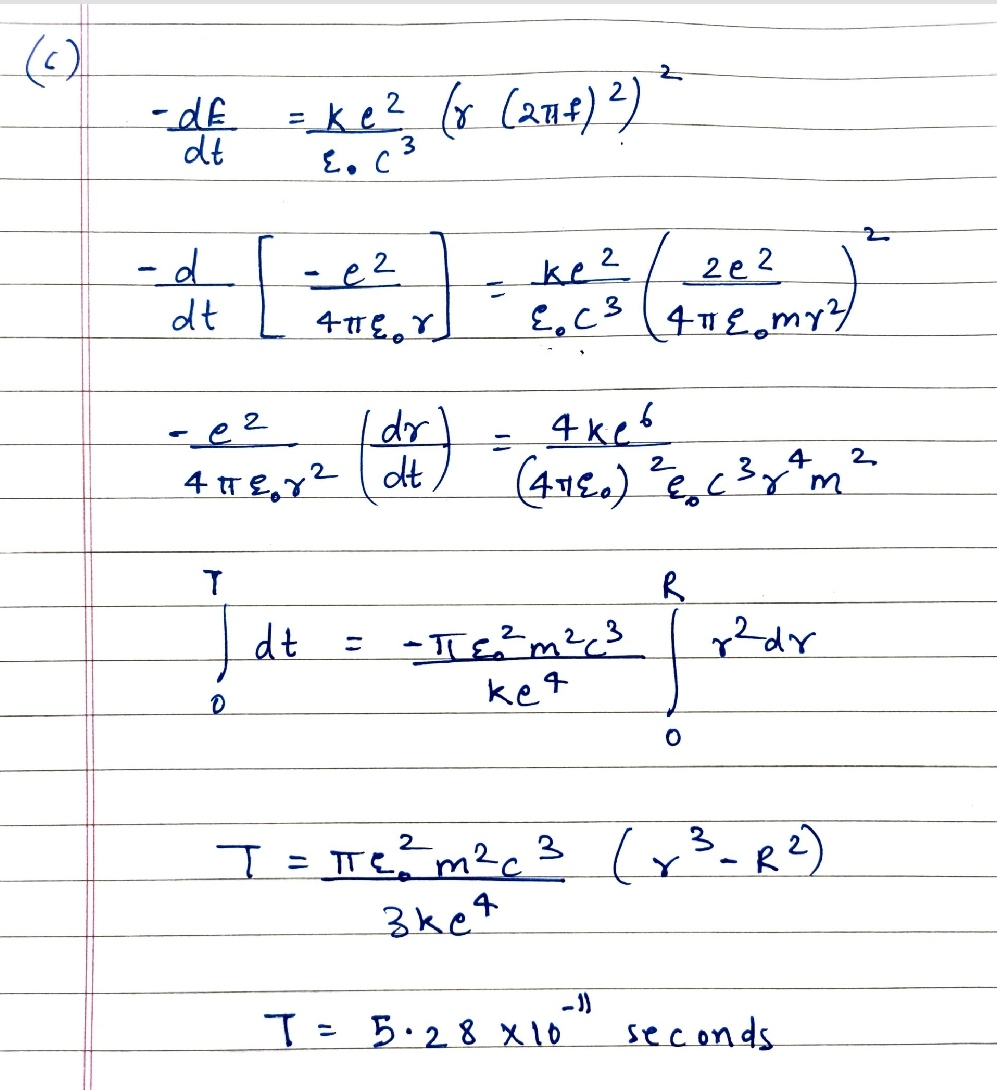- 6 months, 1 week ago

Also why is the acceleration taken as only centripetal acceleration?

- 6 months, 1 week ago

Thanks a lot! But how is the factor $(γ (2πf)^2)=a$ (and what is γ?)

- 6 months, 1 week ago

Oh nevermind, just realised that’s an r

- 6 months, 1 week ago

@Talulah Riley Here is my answer to the problem

- 6 months, 1 week ago